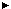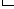# MATHEMATICAL STATISTICS AND PROBABILITY

Mathematical Statistics and Probability: A program that focuses on the mathematical theory underlying statistical methods and their use. Includes instruction in probability theory parametric and non-parametric inference, sequential analysis, multivariate analysis, Bayesian analysis, experimental design, time series analysis, resampling, robust statistics, limit theory, infinite particle systems, stochastic processes, martingales, Markov processes, and Banach spaces.

HomeSearch By DegreeFamily 27Mathematical Statistics and ProbabilityDirectoriesPublic LibrariesOccupations GuideEd DepartmentsSearch EducationLocationNameDegreeAffiliationsCharacteristics